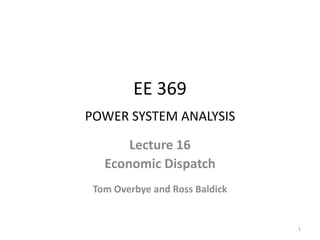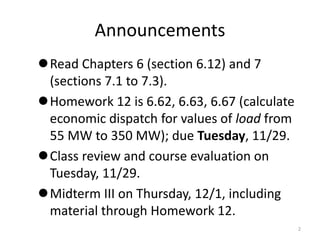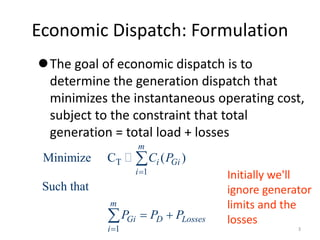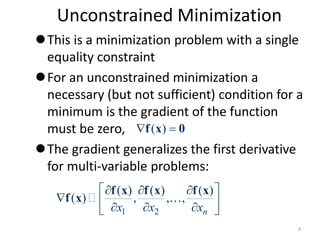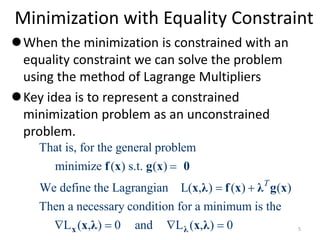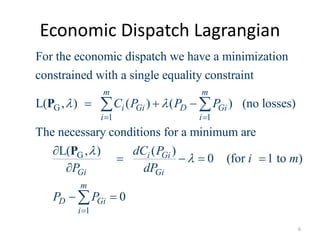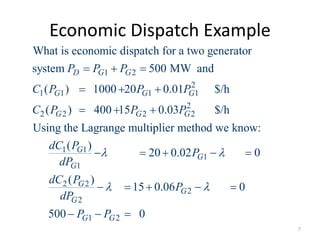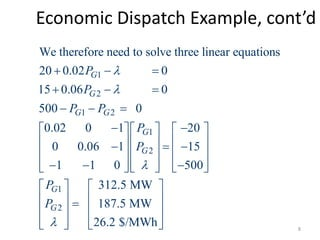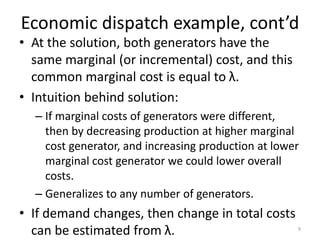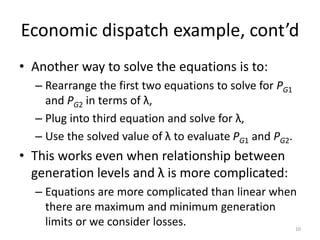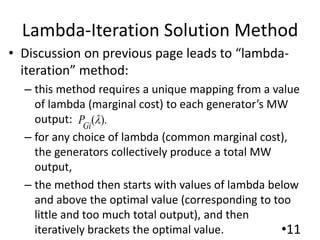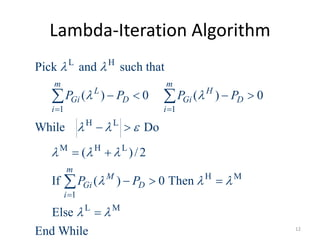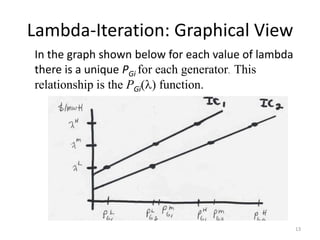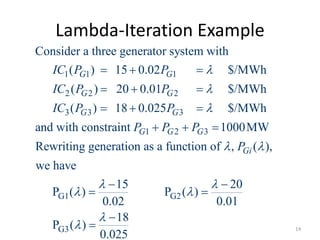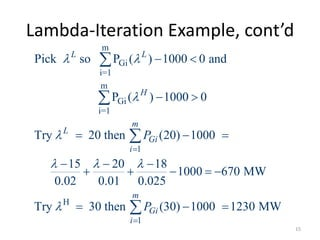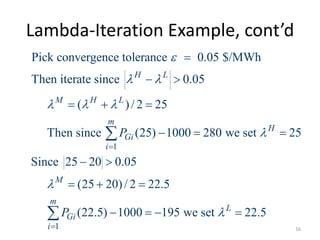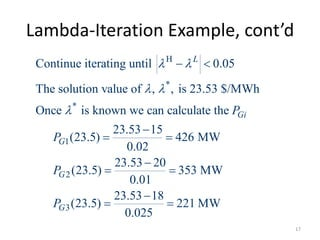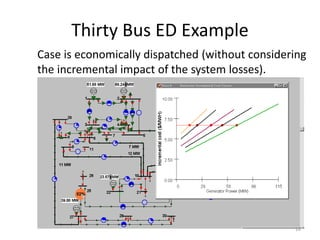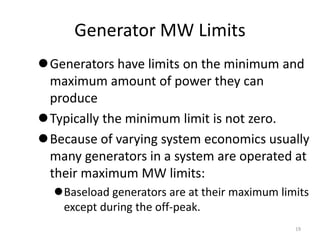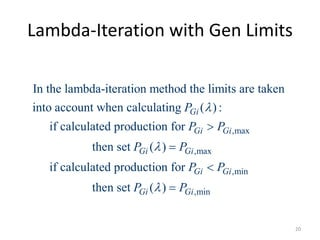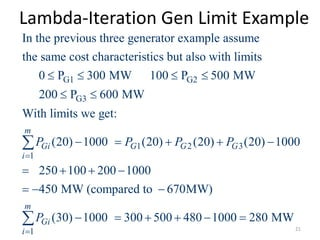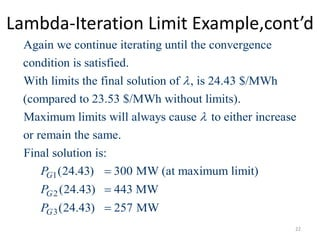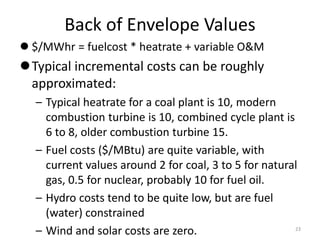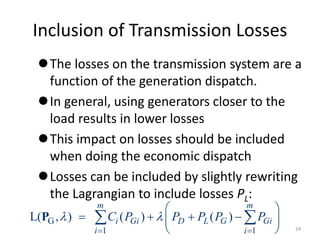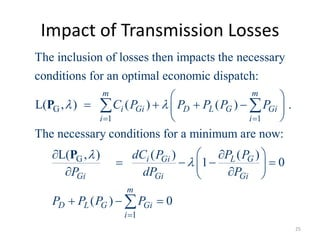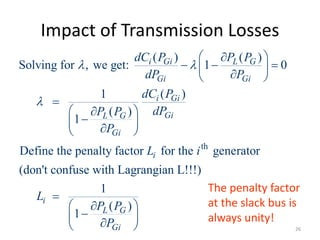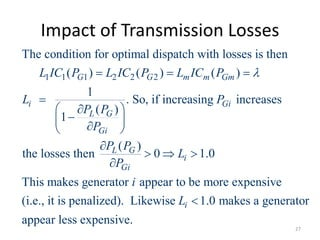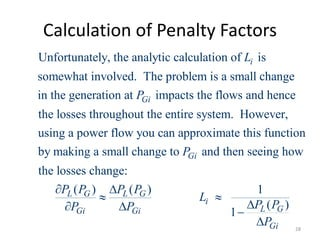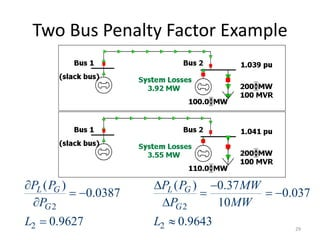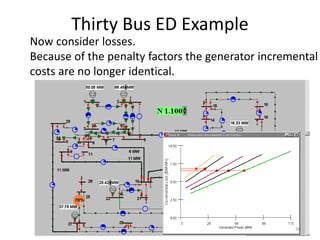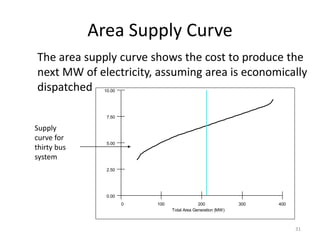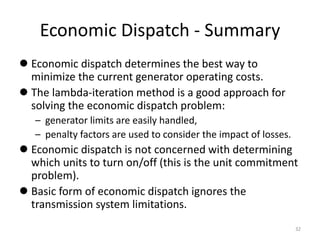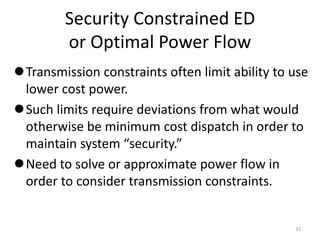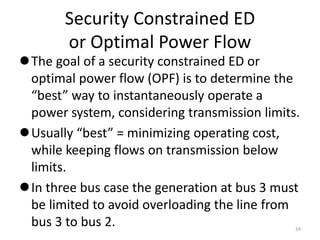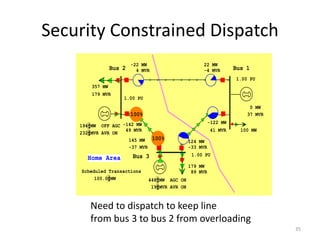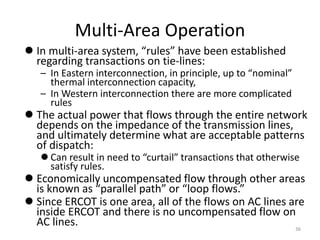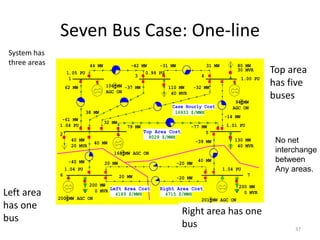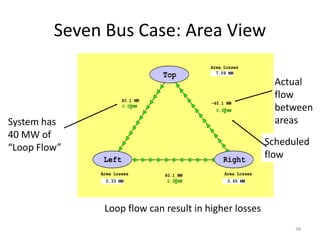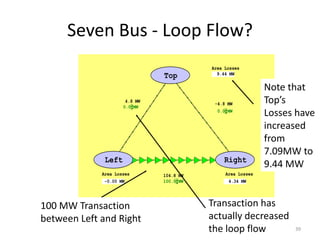1 de 39

### Lecture_16.ppt

1. EE 369 POWER SYSTEM ANALYSIS Lecture 16 Economic Dispatch Tom Overbye and Ross Baldick 1
2. Announcements Read Chapters 6 (section 6.12) and 7 (sections 7.1 to 7.3). Homework 12 is 6.62, 6.63, 6.67 (calculate economic dispatch for values of load from 55 MW to 350 MW); due Tuesday, 11/29. Class review and course evaluation on Tuesday, 11/29. Midterm III on Thursday, 12/1, including material through Homework 12. 2
3. Economic Dispatch: Formulation The goal of economic dispatch is to determine the generation dispatch that minimizes the instantaneous operating cost, subject to the constraint that total generation = total load + losses T 1 1 Minimize C ( ) Such that m i Gi i m Gi D Losses i C P P P P       Initially we'll ignore generator limits and the losses 3
4. Unconstrained Minimization This is a minimization problem with a single equality constraint For an unconstrained minimization a necessary (but not sufficient) condition for a minimum is the gradient of the function must be zero, The gradient generalizes the first derivative for multi-variable problems: 1 2 ( ) ( ) ( ) ( ) , , , n x x x              f x f x f x f x ( )   f x 0 4
5. Minimization with Equality Constraint When the minimization is constrained with an equality constraint we can solve the problem using the method of Lagrange Multipliers Key idea is to represent a constrained minimization problem as an unconstrained problem. That is, for the general problem minimize ( ) s.t. ( ) We define the Lagrangian L( , ) ( ) ( ) Then a necessary condition for a minimum is the L ( , ) 0 and L ( , ) 0 T        x λ f x g x 0 x λ f x λ g x x λ x λ 5
6. Economic Dispatch Lagrangian G 1 1 G For the economic dispatch we have a minimization constrained with a single equality constraint L( , ) ( ) ( ) (no losses) The necessary conditions for a minimum are L( , ) m m i Gi D Gi i i Gi C P P P dC P              P P 1 ( ) 0 (for 1 to ) 0 i Gi Gi m D Gi i P i m dP P P         6
7. Economic Dispatch Example 1 2 2 1 1 1 1 2 2 2 2 2 1 1 1 What is economic dispatch for a two generator system 500 MW and ( ) 1000 20 0.01 \$/h ( ) 400 15 0.03 \$/h Using the Lagrange multiplier method we know: ( ) 20 0.0 D G G G G G G G G G G P P P C P P P C P P P dC P dP              1 2 2 2 2 1 2 2 0 ( ) 15 0.06 0 500 0 G G G G G G P dC P P dP P P              7
8. Economic Dispatch Example, cont’d 1 2 1 2 1 2 1 2 We therefore need to solve three linear equations 20 0.02 0 15 0.06 0 500 0 0.02 0 1 20 0 0.06 1 15 1 1 0 500 312.5 MW 187.5 MW 26.2 \$/MW G G G G G G G G P P P P P P P P                                                          h           8
9. Economic dispatch example, cont’d • At the solution, both generators have the same marginal (or incremental) cost, and this common marginal cost is equal to λ. • Intuition behind solution: – If marginal costs of generators were different, then by decreasing production at higher marginal cost generator, and increasing production at lower marginal cost generator we could lower overall costs. – Generalizes to any number of generators. • If demand changes, then change in total costs can be estimated from λ. 9
10. Economic dispatch example, cont’d • Another way to solve the equations is to: – Rearrange the first two equations to solve for PG1 and PG2 in terms of λ, – Plug into third equation and solve for λ, – Use the solved value of λ to evaluate PG1 and PG2. • This works even when relationship between generation levels and λ is more complicated: – Equations are more complicated than linear when there are maximum and minimum generation limits or we consider losses. 10
11. Lambda-Iteration Solution Method • Discussion on previous page leads to “lambda- iteration” method: – this method requires a unique mapping from a value of lambda (marginal cost) to each generator’s MW output: – for any choice of lambda (common marginal cost), the generators collectively produce a total MW output, – the method then starts with values of lambda below and above the optimal value (corresponding to too little and too much total output), and then iteratively brackets the optimal value. •11 ( ). Gi P 
12. Lambda-Iteration Algorithm L H 1 1 H L M H L H M 1 L M Pick and such that ( ) 0 ( ) 0 While Do ( )/2 If ( ) 0 Then Else End While m m L H Gi D Gi D i i m M Gi D i P P P P P P                                  12
13. Lambda-Iteration: Graphical View In the graph shown below for each value of lambda there is a unique PGi for each generator. This relationship is the PGi() function. 13
14. Lambda-Iteration Example 1 1 1 2 2 2 3 3 3 1 2 3 Consider a three generator system with ( ) 15 0.02 \$/MWh ( ) 20 0.01 \$/MWh ( ) 18 0.025 \$/MWh and with constraint 1000MW Rewriting generation as a function of , ( G G G G G G G G G Gi IC P P IC P P IC P P P P P P                 G1 G2 G3 ), we have 15 20 P ( ) P ( ) 0.02 0.01 18 P ( ) 0.025              14
15. Lambda-Iteration Example, cont’d m Gi i=1 m Gi i=1 1 H 1 Pick so P ( ) 1000 0 and P ( ) 1000 0 Try 20 then (20) 1000 15 20 18 1000 670 MW 0.02 0.01 0.025 Try 30 then (30) 1000 1230 MW L L H m L Gi i m Gi i P P                                 15
16. Lambda-Iteration Example, cont’d 1 1 Pick convergence tolerance 0.05 \$/MWh Then iterate since 0.05 ( )/ 2 25 Then since (25) 1000 280 we set 25 Since 25 20 0.05 (25 20)/ 2 22.5 (22.5) 1000 195 we set 2 H L M H L m H Gi i M m L Gi i P P                                2.5 16
17. Lambda-Iteration Example, cont’d H * * 1 2 3 Continue iterating until 0.05 The solution value of , , is 23.53 \$/MWh Once is known we can calculate the 23.53 15 (23.5) 426 MW 0.02 23.53 20 (23.5) 353 MW 0.01 23.53 18 (23.5) 0.025 L Gi G G G P P P P                221 MW  17
18. Thirty Bus ED Example Case is economically dispatched (without considering the incremental impact of the system losses). 18
19. Generator MW Limits Generators have limits on the minimum and maximum amount of power they can produce Typically the minimum limit is not zero. Because of varying system economics usually many generators in a system are operated at their maximum MW limits: Baseload generators are at their maximum limits except during the off-peak. 19
20. Lambda-Iteration with Gen Limits ,max ,max In the lambda-iteration method the limits are taken into account when calculating ( ) : if calculated production for then set ( ) if calculated production for Gi Gi Gi Gi Gi P P P P P     ,min ,min then set ( ) Gi Gi Gi Gi P P P P    20
21. Lambda-Iteration Gen Limit Example G1 G2 G3 1 2 3 1 In the previous three generator example assume the same cost characteristics but also with limits 0 P 300 MW 100 P 500 MW 200 P 600 MW With limits we get: (20) 1000 (20) (20) (20) 10 m Gi G G G i P P P P              1 00 250 100 200 1000 450 MW (compared to 670MW) (30) 1000 300 500 480 1000 280 MW m Gi i P                21
22. Lambda-Iteration Limit Example,cont’d Again we continue iterating until the convergence condition is satisfied. With limits the final solution of , is 24.43 \$/MWh (compared to 23.53 \$/MWh without limits). Maximum limits will always caus  1 2 3 e to either increase or remain the same. Final solution is: (24.43) 300 MW (at maximum limit) (24.43) 443 MW (24.43) 257 MW G G G P P P     22
23. Back of Envelope Values  \$/MWhr = fuelcost * heatrate + variable O&M Typical incremental costs can be roughly approximated: – Typical heatrate for a coal plant is 10, modern combustion turbine is 10, combined cycle plant is 6 to 8, older combustion turbine 15. – Fuel costs (\$/MBtu) are quite variable, with current values around 2 for coal, 3 to 5 for natural gas, 0.5 for nuclear, probably 10 for fuel oil. – Hydro costs tend to be quite low, but are fuel (water) constrained – Wind and solar costs are zero. 23
24. Inclusion of Transmission Losses The losses on the transmission system are a function of the generation dispatch. In general, using generators closer to the load results in lower losses This impact on losses should be included when doing the economic dispatch Losses can be included by slightly rewriting the Lagrangian to include losses PL: G 1 1 L( , ) ( ) ( ) m m i Gi D L G Gi i i C P P P P P                 P 24
25. Impact of Transmission Losses G 1 1 G The inclusion of losses then impacts the necessary conditions for an optimal economic dispatch: L( , ) ( ) ( ) . The necessary conditions for a minimum are now: L( , ) m m i Gi D L G Gi i i C P P P P P                    P P 1 ( ) ( ) 1 0 ( ) 0 i Gi L G Gi Gi Gi m D L G Gi i dC P P P P dP P P P P P                   25
26. Impact of Transmission Losses th ( ) ( ) Solving for , we get: 1 0 ( ) 1 ( ) 1 Define the penalty factor for the generator (don't confuse with Lagrangian L!!!) 1 ( ) 1 i Gi L G Gi Gi i Gi Gi L G Gi i i L G Gi dC P P P dP P dC P dP P P P L i L P P P                                   The penalty factor at the slack bus is always unity! 26
27. Impact of Transmission Losses 1 1 1 2 2 2 The condition for optimal dispatch with losses is then ( ) ( ) ( ) 1 . So, if increasing increases ( ) 1 ( ) the losses then 0 1.0 This makes generator G G m m Gm i Gi L G Gi L G i Gi L IC P L IC P L IC P L P P P P P P L P                    appear to be more expensive (i.e., it is penalized). Likewise 1.0 makes a generator appear less expensive. i i L  27
28. Calculation of Penalty Factors Unfortunately, the analytic calculation of is somewhat involved. The problem is a small change in the generation at impacts the flows and hence the losses throughout the entire system. However, i Gi L P using a power flow you can approximate this function by making a small change to and then seeing how the losses change: ( ) ( ) 1 ( ) 1 Gi L G L G i L G Gi Gi Gi P P P P P L P P P P P          28
29. Two Bus Penalty Factor Example 2 2 2 2 ( ) ( ) 0.37 0.0387 0.037 10 0.9627 0.9643 L G L G G G P P P P MW P P MW L L             29
30. Thirty Bus ED Example Now consider losses. Because of the penalty factors the generator incremental costs are no longer identical. 30
31. Area Supply Curve 0 100 200 300 400 Total Area Generation (MW) 0.00 2.50 5.00 7.50 10.00 The area supply curve shows the cost to produce the next MW of electricity, assuming area is economically dispatched Supply curve for thirty bus system 31
32. Economic Dispatch - Summary  Economic dispatch determines the best way to minimize the current generator operating costs.  The lambda-iteration method is a good approach for solving the economic dispatch problem: – generator limits are easily handled, – penalty factors are used to consider the impact of losses.  Economic dispatch is not concerned with determining which units to turn on/off (this is the unit commitment problem).  Basic form of economic dispatch ignores the transmission system limitations. 32
33. Security Constrained ED or Optimal Power Flow Transmission constraints often limit ability to use lower cost power. Such limits require deviations from what would otherwise be minimum cost dispatch in order to maintain system “security.” Need to solve or approximate power flow in order to consider transmission constraints. 33
34. Security Constrained ED or Optimal Power Flow The goal of a security constrained ED or optimal power flow (OPF) is to determine the “best” way to instantaneously operate a power system, considering transmission limits. Usually “best” = minimizing operating cost, while keeping flows on transmission below limits. In three bus case the generation at bus 3 must be limited to avoid overloading the line from bus 3 to bus 2. 34
35. Security Constrained Dispatch Bus 2 Bus 1 Bus 3 Home Area Scheduled Transactions 357 MW 179 MVR 194 MW 448 MW 19 MVR 232 MVR 179 MW 89 MVR 1.00 PU -22 MW 4 MVR 22 MW -4 MVR -142 MW 49 MVR 145 MW -37 MVR 124 MW -33 MVR -122 MW 41 MVR 1.00 PU 1.00 PU 0 MW 37 MVR 100% 100% 100 MW OFF AGC AVR ON AGC ON AVR ON 100.0 MW Need to dispatch to keep line from bus 3 to bus 2 from overloading 35
36. Multi-Area Operation  In multi-area system, “rules” have been established regarding transactions on tie-lines: – In Eastern interconnection, in principle, up to “nominal” thermal interconnection capacity, – In Western interconnection there are more complicated rules  The actual power that flows through the entire network depends on the impedance of the transmission lines, and ultimately determine what are acceptable patterns of dispatch:  Can result in need to “curtail” transactions that otherwise satisfy rules.  Economically uncompensated flow through other areas is known as “parallel path” or “loop flows.”  Since ERCOT is one area, all of the flows on AC lines are inside ERCOT and there is no uncompensated flow on AC lines. 36
37. Seven Bus Case: One-line Top Area Cost Left Area Cost Right Area Cost 1 2 3 4 5 6 7 106 MW 168 MW 200 MW 201 MW 110 MW 40 MVR 80 MW 30 MVR 130 MW 40 MVR 40 MW 20 MVR 1.00 PU 1.01 PU 1.04 PU 1.04 PU 1.04 PU 0.99 PU 1.05 PU 62 MW -61 MW 44 MW -42 MW -31 MW 31 MW 38 MW -37 MW 79 MW -77 MW -32 MW 32 MW -14 MW -39 MW 40 MW -20 MW 20 MW 40 MW -40 MW 94 MW 200 MW 0 MVR 200 MW 0 MVR 20 MW -20 MW AGC ON AGC ON AGC ON AGC ON AGC ON 8029 \$/MWH 4715 \$/MWH 4189 \$/MWH Case Hourly Cost 16933 \$/MWH System has three areas Left area has one bus Right area has one bus Top area has five buses 37 No net interchange between Any areas.
38. Seven Bus Case: Area View System has 40 MW of “Loop Flow” Actual flow between areas Loop flow can result in higher losses Area Losses Area Losses Area Losses Top Left Right -40.1 MW 0.0 MW 0.0 MW 0.0 MW 40.1 MW 40.1 MW 7.09 MW 0.33 MW 0.65 MW Scheduled flow 38
39. Seven Bus - Loop Flow? Area Losses Area Losses Area Losses Top Left Right -4.8 MW 0.0 MW 100.0 MW 0.0 MW 104.8 MW 4.8 MW 9.44 MW -0.00 MW 4.34 MW 100 MW Transaction between Left and Right Transaction has actually decreased the loop flow Note that Top’s Losses have increased from 7.09MW to 9.44 MW 39